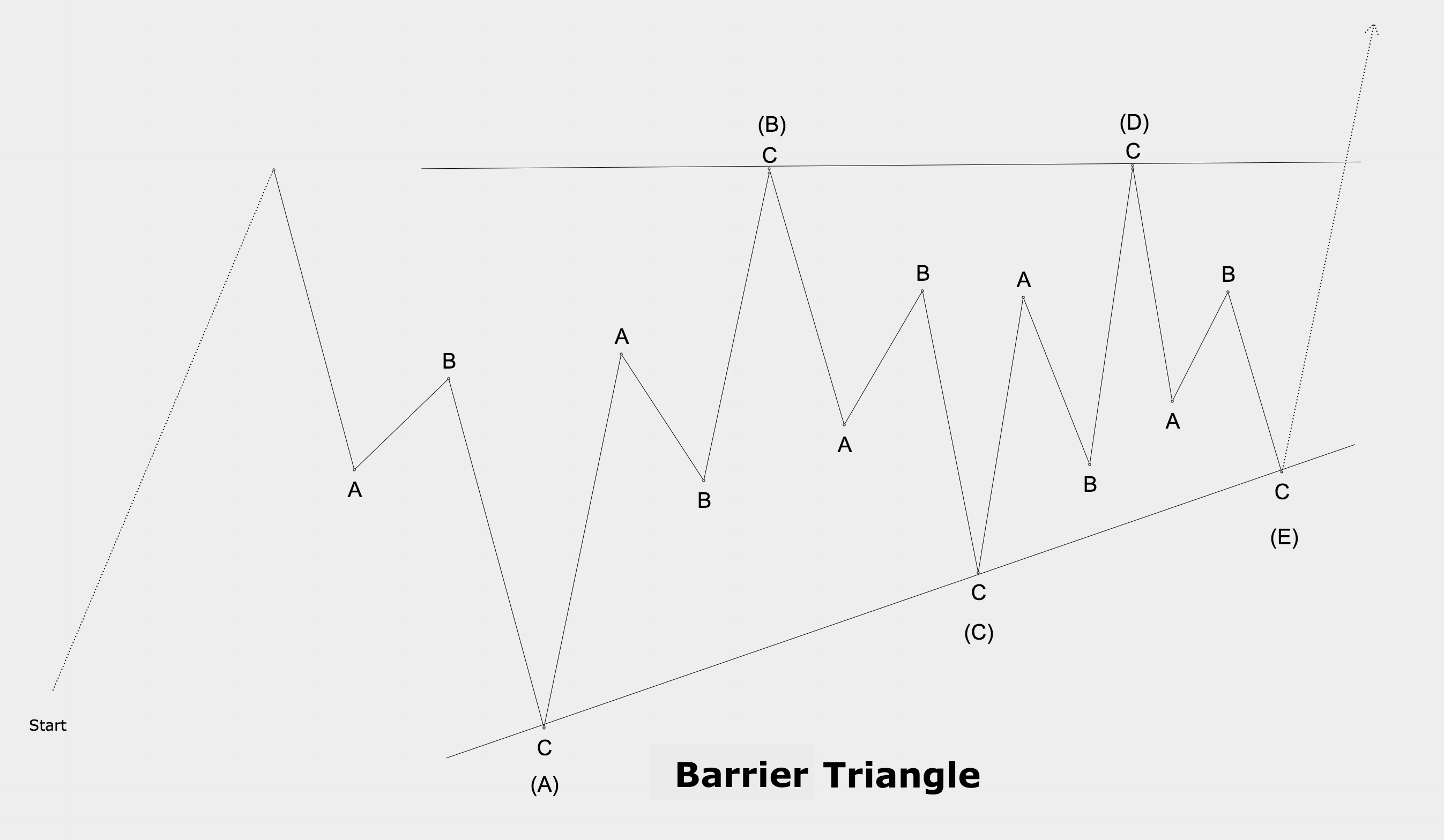### Contracting & Barrier Triangle

Rules

• A triangle subdivides into 5 corrective waves
• Wave C does not move beyond wave A, wave D does not move beyond wave B, and wave E does not move beyond wave C
• A triangle has never more than one complex subwave
• A complex wave within a triangle can be only a zig-zag combination or a triangle
• At least 4 out of 5 waves subdivide into zigzags or zigzag combinations.Guidelines

• Usually wave C,D, or E may subdivide into a barrier or contracting triangle
• A triangle within a triangle results into an „extended“ triangle, which appears to have 9 waves
• Usually, wave C lasts longer and retraces a deeper percentage that the other subways
• Sometimes wave D lasts longer and retraces a deeper percentage that the other subways
• The B-wave of a „running triangle“ ends sometimes beyond the start of the A-wave
• A barrier triangle extends very rarely
• Strength indicators usually contract within a triangle pattern that is not an expanding triangle
• Triangles usually show decreasing volume, interest, and volatility if they are not expanding
• Usually, wave E shows a triangle pattern if a paramount triangle structure extends
• The fifth wave in equities after a triangle resolution is roughly equidistant to the largest triangle fractal
• The fifth wave in commodities after a triangle resolution is usually the longest wave### Expanding Triangle

Rules

Same rules as for contracting and barrier triangles with the following difference:

• Waves B,C, and D retrace between 100%-150% of the previous subwave

Guidelines

Same guidelines as for contracting and barrier triangles with the following difference:

• Waves B,C, and D usually retrace between 105%-130% of the previous subwave
• Expanding triangles almost never extendTechnical Analysis